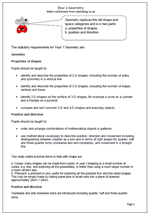# New Maths Curriculum: Year 1 GeometryGeometry replaces the old shape and space categories and is in two parts:

a. properties of shapes
b. position and direction.

The statutory requirements for Year 1 Geometry are:

Properties of shapes

Pupils should be taught to:

•    identify and describe the properties of 2-D shapes, including the number of sides and symmetry in a vertical line

•    identify and describe the properties of 3-D shapes, including the number of edges, vertices and faces

•    identify 2-D shapes on the surface of 3-D shapes, for example a circle on a cylinder and a triangle on a pyramid

•    compare and sort common 2-D and 3-D shapes and everyday objects.

Position and direction

Pupils should be taught to:

•    order and arrange combinations of mathematical objects in patterns

•    use mathematical vocabulary to describe position, direction and movement including distinguishing between rotation as a turn and in terms of right angles for quarter, half and three-quarter turns (clockwise and anti-clockwise), and movement in a straight line.

Two really useful practical items to help with shape are:

a. Cubes: many shapes can be made from cubes. In year 2 keeping to a small number of cubes, e.g. four, and exploring all the possibilities, is better than using a much larger number in a more random way.
b. Pinboard: a pinboard is very useful for exploring all the possible four and five sided shapes. This may be simply made by nailing panel pins or small nails into a piece of plywood approximately 24cm × 24cm.

Position and direction
Clockwise and anti-clockwise turns are introduced including quarter, half and three-quarter turns.

Year 1 Geometry: statutory requirements